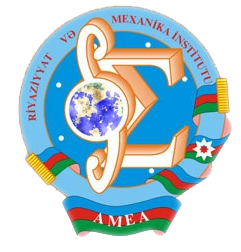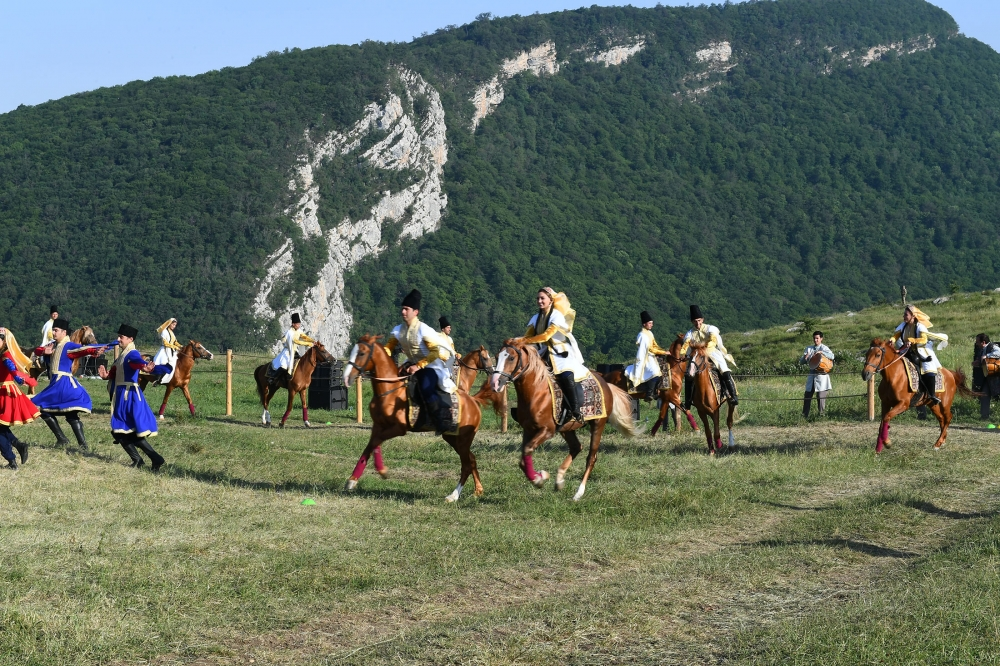Riyaziyyat və Mexanika İnstitutu

## Qarabağ xəbərləri### Şuşada Heydər Əliyev Fondunun təşkilatçılığı ilə “Musiqi irsi və Qarabağ atları Cıdır düzündə” adlı kompozisiya təqdim olunub## Transactions 2004/4/XXIV

 Year: 2004 Volume: 4 Number: XXIV CONTENT Abstract [PDF] The life devoted to science [PDF] 3 MATHEMATICS Akhmedova E.N., Huseynov H.M. Abstract [PDF] On eigenvalues and eigenfunctions of one class of Sturm-Liouville operators with discontinuous coefficients [PDF] 7 Akhundov A.Ya. Abstract [PDF] Determination of a coefficient at higher derivatives in parabolic type semilinear equation. [PDF] 19 Bilalov B.T. , Salmanov V.F. Abstract [PDF] Uniform convergence of biorthogonal series on one system of exponents [PDF] 25 Andriyenko V.A. Abstract [PDF] Pointwise saturation theorems for the Cezaro and Abel means of Fourier series [PDF] 31 Guliyev A.D. Abstract [PDF] On some properties of second order degenerate quasilinear equations [PDF] 35 Guseynov F.B. Abstract [PDF] Asymptotic expansion of solution of Cauchy problem at large time for Barenblatt-Jeltov-Kochina equation [PDF] 43 Iskenderova M.B. Abstract [PDF] On existence of classes of problems with non-summable expansion in root functions. [PDF] 51 Ismailov V.E. Abstract [PDF] On discontinuity of the best approximation of a continuous function [PDF] 57 Jafarov S.Z. Abstract [PDF] On approximation of functions on closed curves with zero angles [PDF] 61 Mamedov I.G. Abstract [PDF] Nonlocal problem for one hyperbolic integro-differential equation with loadings in boundary conditions [PDF] 69 Manafov M.Dzh. Abstract [PDF] Spectral expansion of differential operator with singular potential. [PDF] 79 Mirzoyev R.J. Abstract [PDF] On inner and outer hamming indices of k-valued clones I [PDF] 87 Mirzoyeva K.S. Abstract [PDF] Phragmen-Lindelof type theorem for solutions of the second order elliptic equations non-uniformly degenerating at infinity. [PDF] 95 Mushtagov F.M. Abstract [PDF] The Harnack type inequality for nonnegative solutions of the second order degenerate parabolic equations in divergent form. [PDF] 117 Nabiev I.M. Abstract [PDF] Inverse periodic problem for a diffusion operator. [PDF] 125 Najafov K.M. Abstract [PDF] A boundary value problem with fraction derivatives in the boundary condition for a mixed type second order linear equation with degenerate order [PDF] 131 Namazov G.K., Mehraliyev Y.T. Abstract [PDF] Investigation of classical solution of one-dimensional inverse boundary value problem for semi-linear pseudoparabolic equations of the second order [PDF] 141 Nasibov Sh.M. Abstract [PDF] On some sufficient conditions for the blow-up solutions of the non-linear Ginzburg-Landau-Schrodinger evolution equation [PDF] 149 Novruzov E.B. Abstract [PDF] On the uniqueness of solution of initial boundary value problem for non-linear degenerate equation with non-monotone main part [PDF] 155 Panakhov E.S., Unal Ic. Abstract [PDF] On the determination of the Dirac operator from two spectra [PDF] 159 Rzaev R.M. Abstract [PDF] Singular integral operators on BMO^fi [PDF] 173 Suleymanov S.E. Abstract [PDF] On a mixed problem for wave equation in many-dimensional layer with time derivative in boundary condition. [PDF] 183 Yusifov M.R. Abstract [PDF] Riemann function for the third order pseudoparabolic equations [PDF] 193 MECHANICS Gadjiev V.D., Ahadova S.R. Abstract [PDF] Small parameter method in problem of natural oscillations of nonhomogeneous elastic plate lying on nonhomogeneous foundation [PDF] 199 Guliyev R.S. Abstract [PDF] Dynamic loading of a pile into soil [PDF] 205 Kerimov M.Z., Tagiyev A.E. Abstract [PDF] Distribution of nonstationary temperature difference in the hole-bed system subject to the rate of temperature change of hole wall. [PDF] 209 Namazov S.N. Abstract [PDF] Distribution of nonstationary temperature difference in the hole-bed system subject to the rate of temperature change of hole wall. [PDF] 213 Zamanov A.D. Abstract [PDF] Eigen oscillation of a strip made of composue material with periodic curved structures at pivot support. [PDF] 219
Azərbaycanda COVID-19 ilə bağlı statistika
• Virusa yoluxan

598503

• Sağalan

567385

• Yeni yoluxan

0

• Aktiv xəstə

23114

• Ölüm halı

8004

• Test edilib

5,621,021